15. ÖMG-Kongress Jahrestagung der Deutschen Mathematikervereinigung

16. bis 22. September 2001 in Wien

Sektion 4 - Mathematische Logik, Theoretische Informatik
Donnerstag, 20. September 2001, 17.00, Hörsaal 46

The Puzzle of Transfinite Integers

Endre Kövesi, Wien

Consider the base ten, arabic numerals: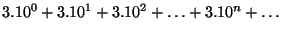also written as 333 ...or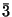. According to set theory the members of the above series are each finite ones, and constitute a least infinite set (of the equinumerical class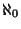) together. So do nonterminating decimals (like: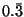). Obviously'' is a finite symbol, representing a classset of digits of the numeral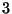''. We can say:islong - digit wise.'' In thislong chain of three-s, each digit has one immediate neighbour. Sinceactually exists according to theory, it has exactly one first and exactly one last member (digit) at the end of infinity''. Clearly, as an individualhas one predecessor (the sum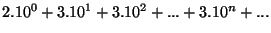) and one successor (the sum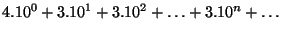). It is a positive whole number (). (So are: 000..., 111..., 222..., ..., 999..., 14142..., 314159..., 271828...). If infinitely long integers exist, what is the total number of these numerals? And what do they represent: finite, or infinite (or both) values? We give here some of the relevant answers and show, how to to integrate the numerical systems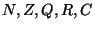of any base and any class in one, simple universal construction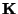. As a result, (while nothing is wrong with the theory of finite sets) Transfinite Set Theory is eliminated.

Zeitplan der Sektion   Tagesübersicht   Liste der Vortragenden